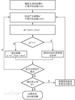Simulated annealing algorithm

SAA

• 模拟退火
模拟退火前言模拟退火的物理知识爬山法模拟退火算法算法流程流程图例子结语
前言
今天昨天+前天+上一周，我学了模拟退火优化算法。想不想知道模拟退火是什么呢？跟我一起来学学吧！
模拟退火的物理知识
在热力学上，退火（annealing）现象指物体逐渐降温的物理现象，温度愈低，物体的能量状态会低；够低后，液体开始冷凝与结晶，在结晶状态时，系统的能量状态最低。大自然在缓慢降温（亦即，退火）时，可“找到”最低能量状态：结晶。但是，如果过程过急过快，快速降温（亦称「淬炼」，quenching）时，会导致不是最低能态的非晶形。
如下图所示，首先（左图）物体处于非晶体状态。我们将固体加温至充分高（中图），再让其徐徐冷却，也就退火（右图）。加温时，固体内部粒子随温升变为无序状，内能增大，而徐徐冷却时粒子渐趋有序，在每个温度都达到平衡态，最后在常温时达到基态，内能减为最小（此时物体以晶体形态呈现）。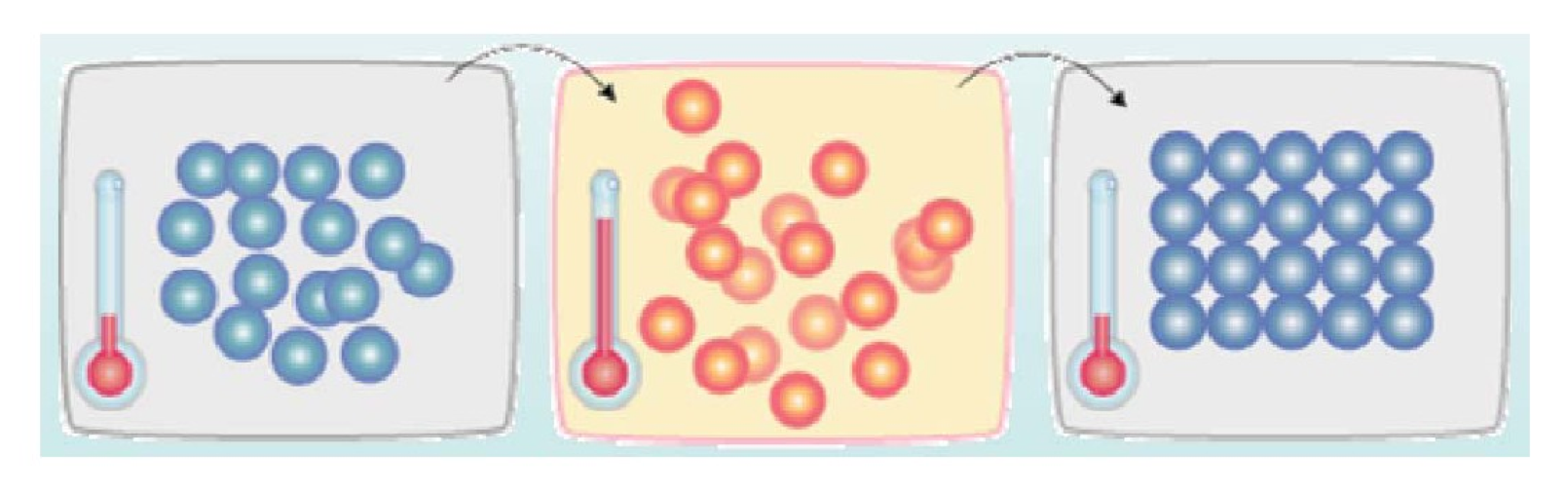似乎，大自然知道慢工出细活：缓缓降温，使得物体分子在每一温度时，能够有足够时间找到安顿位置，则逐渐地，到最后可得到最低能态，系统最安稳。
爬山法
讲模拟退火之前，我们先来讲一个名叫“爬山法”的算法。
爬山法（Hill Climbing，HC）是一种局部择优的贪心搜索算法，其本质上是梯度下降法。
该算法每次从当前的节点开始，与周围的邻接点进行比较：

若当前节点是最大的，那么返回当前节点，作为最大值
若当前节点是最小的，就用最高的邻接点替换当前节点，从而实现向山峰的高处攀爬的目的

如此循环往复，直到达到最高点为止。
但该算法的主要问题是：局部最大，即某个节点会比周围任何一个邻居都高，但只是局部最优解，并非全局最优解。
如下图，在处于当前解时，爬山法搜索到局部最优解后，就会停止搜索，因为在局部最优解这个点，无论向哪个方向小幅度的移动，都无法得到更优解.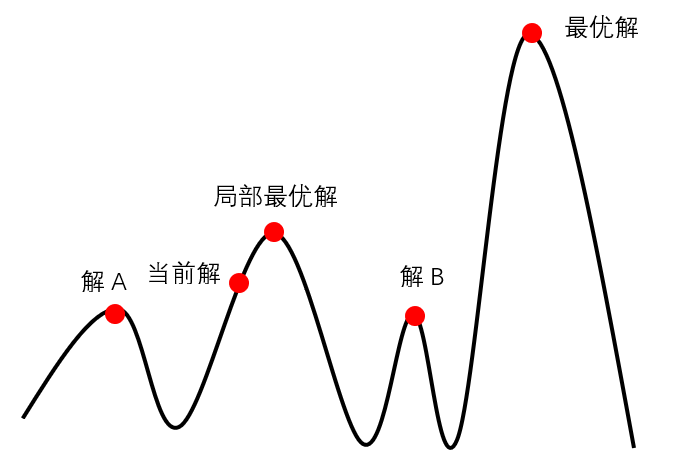此外，其还存在以下两种问题：

高地问题：搜索一旦到达高地，就无法确定搜索最佳方向，会产生随机走动，使得搜索效率降低
山脊问题：搜索可能会在山脊的两面来回震荡，前进步伐很小

当出现以上问题后，只能随机重启爬山算法来解决。
模拟退火算法
如果你对退火的物理意义还是晕晕的，没关系我们还有更为简单的理解方式。想象一下如果我们现在有下面这样一个函数，现在想求函数的（全局）最优解。如果采用Greedy策略，那么从A点开始试探，如果函数值继续减少，那么试探过程就会继续。而当到达点B时，显然我们的探求过程就结束了（因为无论朝哪个方向努力，结果只会越来越大）。最终我们只能找打一个局部最后解B。
模拟退火其实也是一种Greedy算法（贪心算法），但是它的搜索过程引入了随机因素。模拟退火算法以一定的概率来接受一个比当前解要差的解，因此有可能会跳出这个局部的最优解，达到全局的最优解。以上图为例，模拟退火算法在搜索到局部最优解B后，会以一定的概率接受向右继续移动。也许经过几次这样的不是局部最优的移动后会到达B 和C之间的峰点，于是就跳出了局部最小值B。
对于Greedy算法和模拟退火算法，我们可以这样有趣地想：

普通Greedy算法：兔子朝着比现在低的地方跳去。它找到了不远处的最低的山谷。但是这座山谷不一定最低的。这就是普通Greedy算法，它不能保证局部最优值就是全局最优值。

模拟退火：兔子喝醉了。它随机地跳了很长时间。这期间，它可能走向低处，也可能踏入平地。但是，它渐渐清醒了并朝最低的方向跳去。这就是模拟退火。

模拟退火算法从某一较高的温度出发，这个温度称为初始温度，伴随着温度参数的不断下降，算法中的解趋于稳定，但是，可能这样的稳定解是一个局部最优解，此时，模拟退火算法中会以一定的概率跳出这样的局部最优解，以寻找目标函数的全局最优解。如下图中所示，若此时寻找到了$A$点处的解，模拟退火算法会以一定的概率跳出这个解，如跳到了$D$点重新寻找，这样在一定程度上增加了寻找到全局最优解的可能性。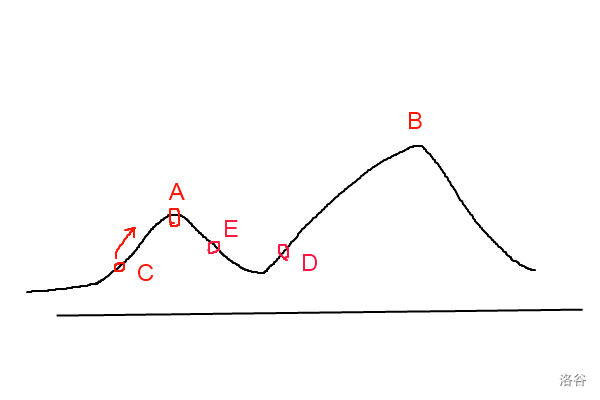算法流程

随机挑选一个单元$k$，并给它一个随机的位移，求出系统因此而产生的能量变化$\Delta E_k$。

若$\Delta E_k\leqslant 0$，该位移可采纳，而变化后的系统状态可作为下次变化的起点；

若$\Delta E_k> 0$，位移后的状态可采纳的概率为：
$P_k=\frac{1}{1+e^{-{\Delta E_k}/{T}}}$
式中T为温度，然后从$(0,1)$区间均匀分布的随机数中挑选一个数$R$，若$R，则将变化后的状态作为下次的起点；否则，将变化前的状态作为下次的起点。
(3)转第(1)步继续执行，直道达到平衡状态为止。
流程图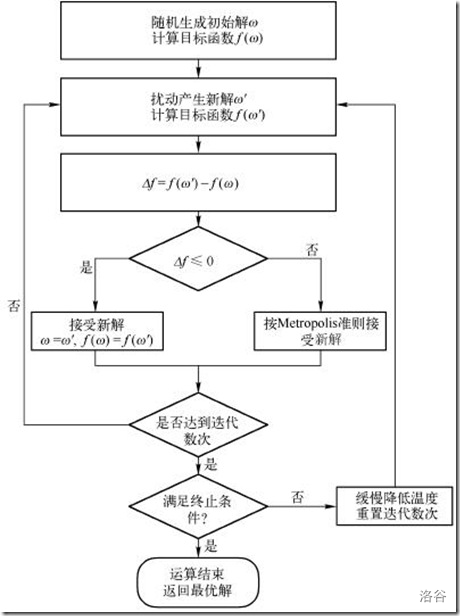例子
通过一个实例来编程演示模拟退火的执行。特别地，我们这里所采用的实例是著名的“旅行商问题”（TSP，Traveling Salesman Problem），它是哈密尔顿回路的一个实例化问题，也是最早被提出的NP问题之一。
TSP是一个最常被用来解释模拟退火用法的问题，因为这个问题比较有名，我们这里不赘言重述，下面直接给出C++实现的代码：
#include <iostream>
#include <string.h>
#include <stdlib.h>
#include <algorithm>
#include <stdio.h>
#include <time.h>
#include <math.h>

#define N     30      //城市数量
#define T     3000    //初始温度
#define EPS   1e-8    //终止温度
#define DELTA 0.98    //温度衰减率

#define LIMIT 1000   //概率选择上限
#define OLOOP 20    //外循环次数
#define ILOOP 100   //内循环次数

using namespace std;

//定义路线结构体
struct Path
{
int citys[N];
double len;
};

//定义城市点坐标
struct Point
{
double x, y;
};

Path bestPath;        //记录最优路径
Point p[N];       //每个城市的坐标
double w[N][N];   //两两城市之间路径长度
int nCase;        //测试次数

double dist(Point A, Point B)
{
return sqrt((A.x - B.x) * (A.x - B.x) + (A.y - B.y) * (A.y - B.y));
}

void GetDist(Point p[], int n)
{
for(int i = 0; i < n; i++)
for(int j = i + 1; j < n; j++)
w[i][j] = w[j][i] = dist(p[i], p[j]);
}

void Input(Point p[], int &n)
{
scanf("%d", &n);
for(int i = 0; i < n; i++)
scanf("%lf %lf", &p[i].x, &p[i].y);
}

void Init(int n)
{
nCase = 0;
bestPath.len = 0;
for(int i = 0; i < n; i++)
{
bestPath.citys[i] = i;
if(i != n - 1)
{
printf("%d--->", i);
bestPath.len += w[i][i + 1];
}
else
printf("%d\n", i);
}
printf("\nInit path length is : %.3lf\n", bestPath.len);
printf("-----------------------------------\n\n");
}

void Print(Path t, int n)
{
printf("Path is : ");
for(int i = 0; i < n; i++)
{
if(i != n - 1)
printf("%d-->", t.citys[i]);
else
printf("%d\n", t.citys[i]);
}
printf("\nThe path length is : %.3lf\n", t.len);
printf("-----------------------------------\n\n");
}

Path GetNext(Path p, int n)
{
Path ans = p;
int x = (int)(n * (rand() / (RAND_MAX + 1.0)));
int y = (int)(n * (rand() / (RAND_MAX + 1.0)));
while(x == y)
{
x = (int)(n * (rand() / (RAND_MAX + 1.0)));
y = (int)(n * (rand() / (RAND_MAX + 1.0)));
}
swap(ans.citys[x], ans.citys[y]);
ans.len = 0;
for(int i = 0; i < n - 1; i++)
ans.len += w[ans.citys[i]][ans.citys[i + 1]];
cout << "nCase = " << nCase << endl;
Print(ans, n);
nCase++;
return ans;
}

void SA(int n)
{
double t = T;
srand((unsigned)(time(NULL)));
Path curPath = bestPath;
Path newPath = bestPath;
int P_L = 0;
int P_F = 0;
while(1)       //外循环，主要更新参数t，模拟退火过程
{
for(int i = 0; i < ILOOP; i++)    //内循环，寻找在一定温度下的最优值
{
newPath = GetNext(curPath, n);
double dE = newPath.len - curPath.len;
if(dE < 0)   //如果找到更优值，直接更新
{
curPath = newPath;
P_L = 0;
P_F = 0;
}
else
{
double rd = rand() / (RAND_MAX + 1.0);
//如果找到比当前更差的解，以一定概率接受该解，并且这个概率会越来越小
if(exp(dE / t) > rd && exp(dE / t) < 1)
curPath = newPath;
P_L++;
}
if(P_L > LIMIT)
{
P_F++;
break;
}
}
if(curPath.len < bestPath.len)
bestPath = curPath;
if(P_F > OLOOP || t < EPS)
break;
t *= DELTA;
}
}

int main(int argc, const char * argv[]) {

freopen("TSP.data", "r", stdin);
int n;
Input(p, n);
GetDist(p, n);
Init(n);
SA(n);
Print(bestPath, n);
printf("Total test times is : %d\n", nCase);
return 0;
}


我这有一道不会的题目：

求解函数最小值问题：

$F\left ( x \right )=6x^7+8x^6+7x^3+5x^2-xy$

其中，$0\leq x\leq 100$，输入任意$y$值，求$F(x)$的最小值。

各位大佬们，能帮帮我这个蒟蒻吗？
结语
好了，我今天的讲解就结束了，如果我的公式有问题请联系我改正，谢谢大家！


展开全文...